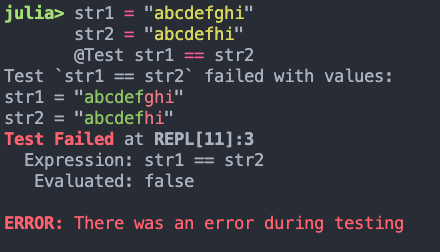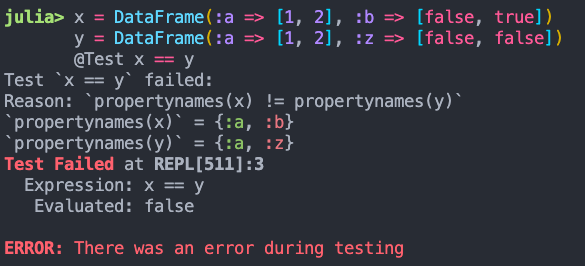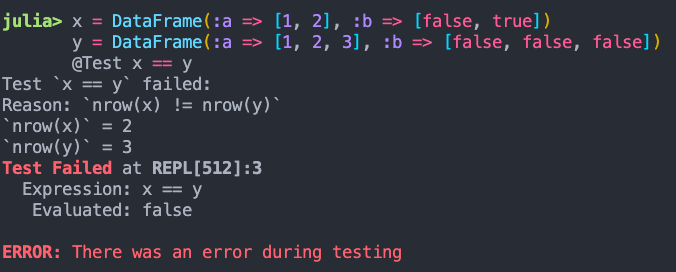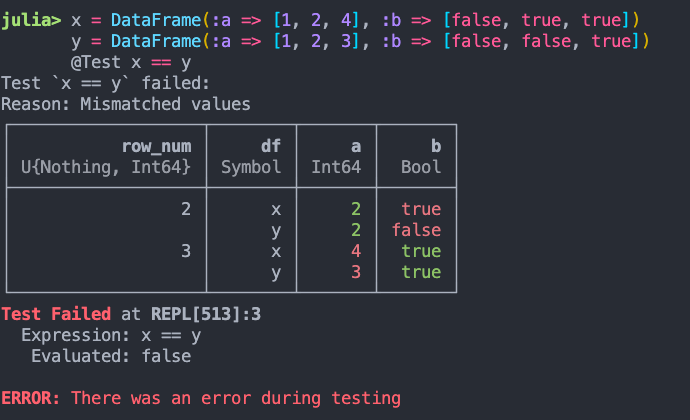# [ANN] TestingUtilities.jl

Hey all,

I’m happy to announce TestingUtilities.jl, which provides macros to (hopefully) make your package testing experience a lot smoother. This package is set to be registered in the `General` registry in two days as of writing this post.

If you’ve ever made a number of changes to your code which cause your tests to fail, Julia’s excellent `Test` module makes it easy to which tests have failed but not specifically why those tests have failed.

The `@Test` macro (note the capitalization) in this package helps to alleviate this problem by displaying relevant values that caused your test to fail, even if the test expression is somewhat complicated
e.g., evaluating

``````inner_comparison_func(a,b) = a == 2*b
g = x->2x
a = 2
b = 1
@Test inner_comparison_func(a, g(b))
``````

outputs

``````Test `inner_comparison_func(a, g(b))` failed with values:
a = 2
`g(b)` = 6
b = 3

Test Failed at REPL:1
Expression: inner_comparison_func(a, g(b))
Evaluated: false

ERROR: There was an error during testing
``````

This macro works by building up a computational graph of the expression until it finds `Symbols` that are the input values to this test. If the test fails, it displays the input values (as well as the value of the “top-level” args + kwargs from the test expression) that caused said test to fail.

Currently not all Julia syntactical constructions are supported, but if your test expressions are sufficiently simple, this macro should help you reason about why things have gone awry from the output of your logs.

When run from an interactive Julia session, the input variables which cause the test failures are set in the `Main` module. This can be helpful when, for instance, you’re evaluating an entire `@testset` at a time with multiple `@Test` expressions, some of which are failing.

The `@test_cases` macro allows one to compactly validate a test expression on a number of identically structured test case instances. Similar to `@Test`, it will output the test case values that cause the each expression to fail, if they do, in fact, fail.

``````@test_cases begin
a | b | output
1 | 2 | 3
1 | 2 | 4
0 | 0 | 1
0 | 1 | 2
@test a + b == output
end
``````

Outputs

``````Test Failed at REPL:1
Expression: a + b == output
Evaluated: false

Test `a + b == output` failed with values:
------
`a + b` = 3
output = 4
a = 1
b = 2
ERROR: There was an error during testing
``````

`@test_cases` halts execution on the first test case that causes a failure when invoked on its own. To capture all failing test instances, run it within a `@testset`, e.g.,

``````@testset begin
@test_cases begin
a | b | y
1 | 2 | 3
1 | 2 | 4
0 | 0 | 1
@test a + b == y
@test b^2 + 1 == y
end
end
``````

Outputs:

``````Test `a + b == y` failed with values:
------
`a + b` = 3
y = 4
a = 1
b = 2
------
`a + b` = 0
y = 1
a = 0
b = 0
Test `b ^ 2 + 1 == y` failed with values:
------
`b ^ 2 + 1` = 5
y = 3
a = 1
b = 2
------
`b ^ 2 + 1` = 5
y = 4
a = 1
b = 2
``````

There is also alternative equivalent syntax for writing test cases, if the `|`-delimited version results in expressions that are too difficult to read.

``````@testset begin
@test_cases begin
input1 | input2 | output
(input1 = 5, input2 = "aabcdddfasdfasdfasdfasdf", output = false)
input1 => 0,
input2 => "abcdefgh", output => false
(input1 = 5, input2 = "aaaaa", output = true)
@test (length(input2) == length(input1)) == output
end
end
``````

Outputs

``````test set: Test Failed at REPL:2
Expression: (length(input2) == length(input1)) == output
Evaluated: false

Test `(length(input2) == length(input1)) == output` failed with values:
------
`length(input2) == length(input1)` = false
output = true
input1 = 5
input2 = "aaaaa"
Test Summary: | Pass  Fail  Total  Time
test set      |    2     1      3  0.1s
ERROR: Some tests did not pass: 2 passed, 1 failed, 0 errored, 0 broken.
``````

Not all of Julia’s syntax is currently handled in the expression parsing, but if your test expressions are sufficiently simple, these macros should handle extracting the relevant `Symbol`s and `Expr`s to output on failure.

If you have any questions or comments, please let me know below or on the Github repo. Happy testing!

15 Likes

I like to do

``````for val in (x, y, z)
@test munge(x)
end
``````

But I don’t do this because I can’t tell whether `x`, `y`, or `z` failed. Maybe this package would solve this problem.

for that I use

``````@testset "\$val" for val in (x, y, z)
@test munge(val)
end
``````
18 Likes

Look forward to this.
how about a shorter name TestUtils.jl? TestPlus?

## Version 1.3.0

Version 1.3.0 is out now and brings some convenient features for failing equality tests, e.g., `@Test x == y` or `@Test isequal(x,y)`. When `x` and `y` have specific types listed below, `TestingUtilities` will print out a nicely formatted message about why specifically these two quantities are not equal (similar in spirit to WhyNotEqual.jl).

### Strings

If `x` and `y` are two `String`s, the failing test will display the common prefix of `x` and `y` in green and the subsequently differing suffix in red, e.g.,If you’re unable to distinguish between red & green text, or you just want to change how the differences are displayed, you can invoke `TestingUtilities.set_show_diff_styles`, which accepts any keyword arguments also accepted by `Base.printstyled`, e.g.,

### DataFrames (Julia 1.9+ only)

If `x` and `y` are two `DataFrames`, the failing test will display the reason that `x` and `y` are not equal, e.g.,

• `x` and `y` have differing column names• `x` and `y` have the same column names but differing number of rows• `x` and `y` have the same column names and number of rows, but differing valuesAs the `DataFrames` package are loaded via package extensions, this feature requires at least Julia 1.9.

6 Likes

# Version 1.4.0

Version 1.4.0 of TestingUtilities.jl is out now, adding the `@test_eventually` macro which is used to test that a given test expression eventually returns true (i.e., passes within a prescribed timeout). You can use it to test, for instance, that blocking expressions eventually return within a given time limit or will throw a `TestTimedOutException` in your test set instead. e.g.,

``````@testset "Timing Out Test" begin
done = Ref(false)
f = (done)->(while !done[]; sleep(0.1) end; true)

# Times out after 1s, checking every 10ms that a value has not returned
@test_eventually timeout=1s sleep=10ms f(done)

# Test passes within the allotted timeout
g = @async (sleep(0.3); done[] = true)
@test_eventually timeout=1s sleep=10ms f(done)
end
``````

Sample output:

``````    Test `f(done)` failed:
Reason: Test took longer than 1000 milliseconds to pass
Values:
done = Base.RefValue{Bool}(false)
Timing Out Test: Error During Test at REPL:6
Test threw exception
Expression: f(done)

Test Summary:   | Pass  Error  Total  Time
Timing Out Test |    1      1      2  1.5s
ERROR: Some tests did not pass: 1 passed, 0 failed, 1 errored, 0 broken.
``````
1 Like

# Version 1.6

Version 1.6 of TestingUtilities.jl is out now.

## DataFrame Printing

You can now specify the maximum number of rows/columns shown package-wide for each dataframe by calling `TestingUtilities.set_show_df_opts(; max_num_rows::Int, max_num_cols::Int)` or `TestingUtilities.set_show_diff_df_options(; max_num_rows::Int, max_num_cols::Int)`, used when showing DataFrame values in non-equality tests or equality tests, respectively.

Rows/columns that exceed this setting are truncated as “…”, e.g.,

``````julia> using TestingUtilities, DataFrames
julia> df = DataFrame( (Symbol("a\$i") => (i:i+10) for i in 1:10)... );
julia> TestingUtilities.set_show_df_opts(; max_num_rows=2, max_num_cols=2)
julia> @Test df[1,:a1] == 2
Test `df[1, :a1] == 2` failed:
Values:
`df[1, :a1]` = 1
df = ┌───────┬───────┬───┐
│    a1 │    a2 │ … │
│ Int64 │ Int64 │   │
├───────┼───────┼───┤
│     1 │     2 │ ⋯ │
│     2 │     3 │   │
│     ⋮ │     ⋮ │   │
└───────┴───────┴───┘
Test Failed at REPL:1
Expression: df[1, :a1] == 2
Evaluated: false

ERROR: There was an error during testing
``````

## Generic Struct Diff Printing

When evaluating a failing test of the form `x == y` or `isequal(x, y)`, this package shows the differing values of `x::T` and `y::T` in particular ways, depending on the type `T`. When `T` is a generic struct type, the values of each field of `x` and `y` where `!(getfield(x,k) == getfield(y,k))` are shown, as well as each of their differing subfields, e.g.,

``````julia> struct ShowDiffChild1_1
x::String
y::Int
end

julia> struct ShowDiffChild1_2
y::Bool
z::Float64
end

julia> struct ShowDiffChild1_3
a::Vector{Int}
end

julia> struct ShowDiffChild1
key1::Union{ShowDiffChild1_1, ShowDiffChild1_2}
key2::Dict{String, Any}
end

julia> struct ShowDiffChild2
key3::Symbol
end

julia> struct ShowDiffParent
child::Union{ShowDiffChild1, ShowDiffChild2}
end

julia> x = ShowDiffParent(ShowDiffChild1(ShowDiffChild1_1("abc", 0), Dict{String,Any}())); y = ShowDiffParent(ShowDiffChild1(ShowDiffChild1_1("abc", 1), Dict{String,Any}()));

julia> @Test x == y
Test `x == y` failed:
Differing fields between `x` and `y`:

x::ShowDiffParent = ShowDiffParent(ShowDiffChild1(ShowDiffChild1_1("abc", 0), Dict{String, Any}()))
y::ShowDiffParent = ShowDiffParent(ShowDiffChild1(ShowDiffChild1_1("abc", 1), Dict{String, Any}()))

x.child::ShowDiffChild1 = ShowDiffChild1(ShowDiffChild1_1("abc", 0), Dict{String, Any}())
y.child::ShowDiffChild1 = ShowDiffChild1(ShowDiffChild1_1("abc", 1), Dict{String, Any}())

x.child.key1::ShowDiffChild1_1 = ShowDiffChild1_1("abc", 0)
y.child.key1::ShowDiffChild1_1 = ShowDiffChild1_1("abc", 1)

x.child.key1.y::Int64 = 0
y.child.key1.y::Int64 = 1
Test Failed at REPL:1
Expression: x == y
Evaluated: false
``````
5 Likes